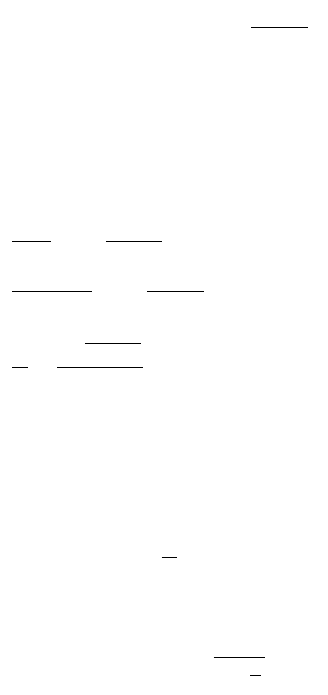EXAMPLE 22.14. Using this equation, calculate the conversion factor of
the 5 1/4s of 02/15/29 U.S. T-bond deliverable into the Chicago Board of Trade’s
U.S. T-bond futures contract expiring in March of 2000.
C = 0.0525
N = 28 (March 1, 2000, through March 1, 2028; remember, full years)
X = 9 months (March 1 through February 15, rounded down from 11 months
to the number of months in the nearest full quarter, which in this case is
9 months.
UNDERSTANDING THE EQUATIONS FOR
COMPUTING CONVERSION FACTORS
Like many ﬁxed-income mathematics equations, these conversion factor equa-
tions appear more fearsome than they actually are. We’ll look at the one for
the 10-year Treasury notes and explain the terms. The other three equations
are comparable.
Here is the equation for the 10-year T-note:
where
CF = Conversion factor
C = Annual coupon on \$100 face amount (in decimal form)
N = Complete years to call or maturity
X = Number of months in excess of the whole N (rounded down to com-
plete quarters).
The annual interest rate (yield) is 6%, or 0.06 in decimal form, compounded
semiannually. The true yield is therefore 3%, or 0.03, semiannually.
X is rounded down to complete quarters, so X can equal only 3, 6, or 9. If
X = 9, then set X = 3 and increase the 2N factor to 2N + 1. Similarly, if X = 6,
set X = 0 and increase 2N to 2N + 1. You can see that if X is 6 or 9, we increase
the number of full periods by 1 (one-half year) and reduce X by 6 (months).
CF
CC C X
XNN
=+¥-
È
Î
Í
˘
˚
˙
+
È
Î
Í
˘
˚
˙
-
()
1
103
2006
1
1
103
1
103
2
6
6
6
22
.
.
..
CF
CF
CF
=+¥-
È
Î
Í
˘
˚
˙
+
È
Î
Í
˘
˚
˙
-
()
=+¥
[]
+
[]
-
=
1
103
0 0525
2
0 0525
006
1
1
103
1
103
0 0525
2
63
6
0 98533 0 0263 0 875 0 814528 0 18547193 0 013125
0 8977
3
6
57 57
.
..
.
..
.
.... . .
.
302 Futures ContractsAll calculations for this conversion factor are to a quarter. Note that all calcu-
lations for 2-year and 5-year notes are rounded down to the next whole month,
so all calculations for these conversion factors are to a whole month. These all
differ from the standard bond price calculation, which is to an exact day, as
shown in Chapter 6, Equation 6.1.
The conversion factor applies to a unit amount (1). The conversion factor
is therefore multiplied by the actual par amount of the trade and by the futures
contract price, to determine the actual cash for the principal amount of the
bonds delivered. Accrued interest is added as well, as usual for bond trades.
The last term inside the outer square brackets is the present
value of the ﬁnal maturity amount. It shows the future value (1) discounted
at 3% for 2N semiannual periods. If the original calculation for X gave X = 6
or X = 9, then X was reduced by 6 and 2N increased to 2N + 1.
The middle term inside the outer square brackets is the present value of the
annuity of coupon payments, as displayed in Equation 5.6 in Chapter 5 and
as shown in the following development:
Once more, if the original value was X = 6 or X = 9, X was reduced by 6
and 2N increased to 2N + 1.
We have now the two parts of the standard bond price calculation.
The seller of the bond is entitled to the accrued interest on the bond. The
first term inside the outer square bracket, , is the accrued interest due for
one-half year. If the note is priced on an even semiannual period, this is the
accrued interest.
The term outside the outer square brackets, , equals 1 if the con-
version factor is priced on a semiannual period, because X = 0. If the conver-
sion factor is priced to a quarterly period, the factors inside the outer square
brackets are discounted back to the previous semiannual period at a 3% semi-
annual rate.
1
103
6
.
X
C
2
È
Î
Í
˘
˚
˙
C
C
C
N
N
N
006
1
1
103
2003
1
1
103
2
1
1
103
003
2
2
2
.
.
.
.
.
.
¥-
È
Î
Í
˘
˚
˙
È
Î
Í
˘
˚
˙
=
¥
()
¥-
È
Î
Í
˘
˚
˙
È
Î
Í
˘
˚
˙
=
¥
-
È
Î
Í
Í
Í
˘
˚
˙
˙
˙
È
Î
Í
Í
Í
˘
˚
˙
˙
˙
+
È
Î
Í
˘
˚
˙
Ê
Ë
ˆ
¯
1
103
2
.
N
Understanding the Equations for Computing Conversion Factors 303

Get Fixed Income Mathematics now with the O’Reilly learning platform.

O’Reilly members experience live online training, plus books, videos, and digital content from nearly 200 publishers.